Courses

# Test: Shear Strength Of Soil - 1

## 10 Questions MCQ Test Topicwise Question Bank for GATE Civil Engineering | Test: Shear Strength Of Soil - 1

Description
This mock test of Test: Shear Strength Of Soil - 1 for Civil Engineering (CE) helps you for every Civil Engineering (CE) entrance exam. This contains 10 Multiple Choice Questions for Civil Engineering (CE) Test: Shear Strength Of Soil - 1 (mcq) to study with solutions a complete question bank. The solved questions answers in this Test: Shear Strength Of Soil - 1 quiz give you a good mix of easy questions and tough questions. Civil Engineering (CE) students definitely take this Test: Shear Strength Of Soil - 1 exercise for a better result in the exam. You can find other Test: Shear Strength Of Soil - 1 extra questions, long questions & short questions for Civil Engineering (CE) on EduRev as well by searching above.
QUESTION: 1

### Which one of the following conditions is valid in case of unconfined compression test in comparison to tri-axial test?

Solution:

Unconfined compression test is a special case of tri-axial test in which the confining pressure is zero. This test may be conducted on undisturbed or remoulded cohesive soils. It cannot be conducted on coarse-grained soils such as sands and gravels as these cannot stand without lateral support.

QUESTION: 2

### Which one of the following is the appropriate tri-axial test to assess the immediate stability of an unloading prolfem, such as an excavation of a clay slope?

Solution:

For problems of short term-stability of foundations: excavations and earth dams UU (Unconsolidated, Undrained) tests are appropriate. For problems of long-term stability either UU test or CD test is appropriate, depending upon the drainage conditions of soil.

QUESTION: 3

### In a tri-axial test at failure, major principal stress was 180 kPa, minor principal stress was 100 kPa, and pore pressure was 20 kPa. The sine of the angle of shearing resistance of the sandy soil tested is

Solution:

Effective minor principal stress.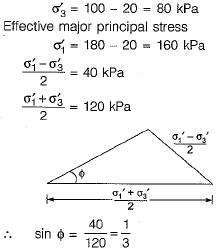QUESTION: 4

The relationship between a soil cohesion c and unconfined compressive strength qu is given as

Solution:
QUESTION: 5

In tri-axial compression test, the deviator stress is given by

Solution:
QUESTION: 6

For saturated soil, Skempton’s B-parameter is

Solution:

Skempton’s B-parameter is given by,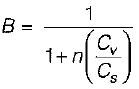Cv is volume compressibility of pore fluid under isotropic conditions,
Cs is the coefficient of compressibility of the soil skeleton;n is porosity
In a fully saturated soil, the compressibility of the pore water (Cv) is negligible compared with the compressibility of the soil mass (Cs). Therefore, the ratio (Cv/Cs) tends to zero and the coefficient B becomes equal to unity.
In a partially saturated soil, the compressibility of the air in the voids Is high. The ratio (Cv/Cs) has a value greater than unity, and, therefore, the pore pressure coefficient B has a value of less than unity.

QUESTION: 7

In an unconfined compression test on a clay specimen of initial volume V and length L, the area of cross-section at failure is

Solution:

As the sample is sheared, its length decreases and the diameter increases. The cross-sectional area A at any stage during shear can be determined assuming that the sample remains cylindrical in shape. Let ΔL0 be the change in length and ΔV0 be the change in volume. The volume of the specimen at any stage is given by V0 ± ΔV0.
Therefore, A(L0 - ΔL0) = V0 ± ΔV0
or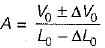For an undrained test as the volumetric change
(ΔV0) is zero therefore,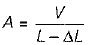QUESTION: 8

The stresses responsible for mobilisation of shearing strength of soil is

Solution:

The shear strength of a soil is its maximum resistance to shear stress just before the failure. Shear stress develop when soils are subjected to compression.
Terzaghi established that the normal stresses which control the shear strength of a soil are the effective stresses and not the total stresses. In terms of effective stresses shear strength is written as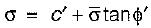where c' and ϕ' are the cohesion intercept and the angle of shearing resistance in terms of the effective engineering stress.

QUESTION: 9

In a direct shear test, the shear stress and normal stress on a dry sand sample at failure are 0.6 kg/cm2 and 1 kg/cm2 respectively. The angle of internal friction of the sand will be nearly:

Solution:

Shear strength of soil (τ) is related with its angie of internal friction (ϕ) by,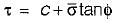c is cohesion; for sand c = 0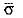is effective normal stress; for dry sand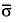= σ normal stress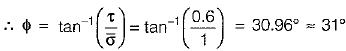QUESTION: 10

A dense dry sand sample under tri-axial test has the confining pressure of 20 t/m2. The angle of internal! friction is 35°. The sample will fail at normal pressure of about:

Solution:

σn = σ3(1 + sin βman)
βman ≌ ϕ
∴ σn = 20 x (1 + sin 35°)
= 31.47 t/m2

• Shear